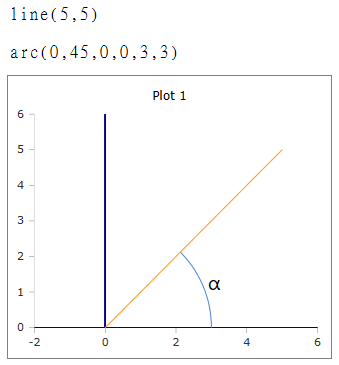# Arc Function

Draw a circular arc

### Description

The $$Arc$$ function draws a circular arc in a plot box.

### Syntax

Arc (start, len, x, y, xr, yr)

Arc (start, len, x, y, xr, yr, id)

### Parameters

start

The angle of the vector where the arc begins. The value must be specified in degrees. The zero position is the positive x-axis, that is, to the right of the center of the arc.

len

The length of the arc as an angle in degrees, starting from the starting point. The arc is drawn left-handed from the starting point.

x

x position of the center of the arc

y

y position of the center of the arc

xr

Radius of the x-axis of the arc.

yr

Radius of the y-axis of the arc.

### Optional Parameters

id

Id number of the plot box, if more than one box is open on the worksheet.

### Example